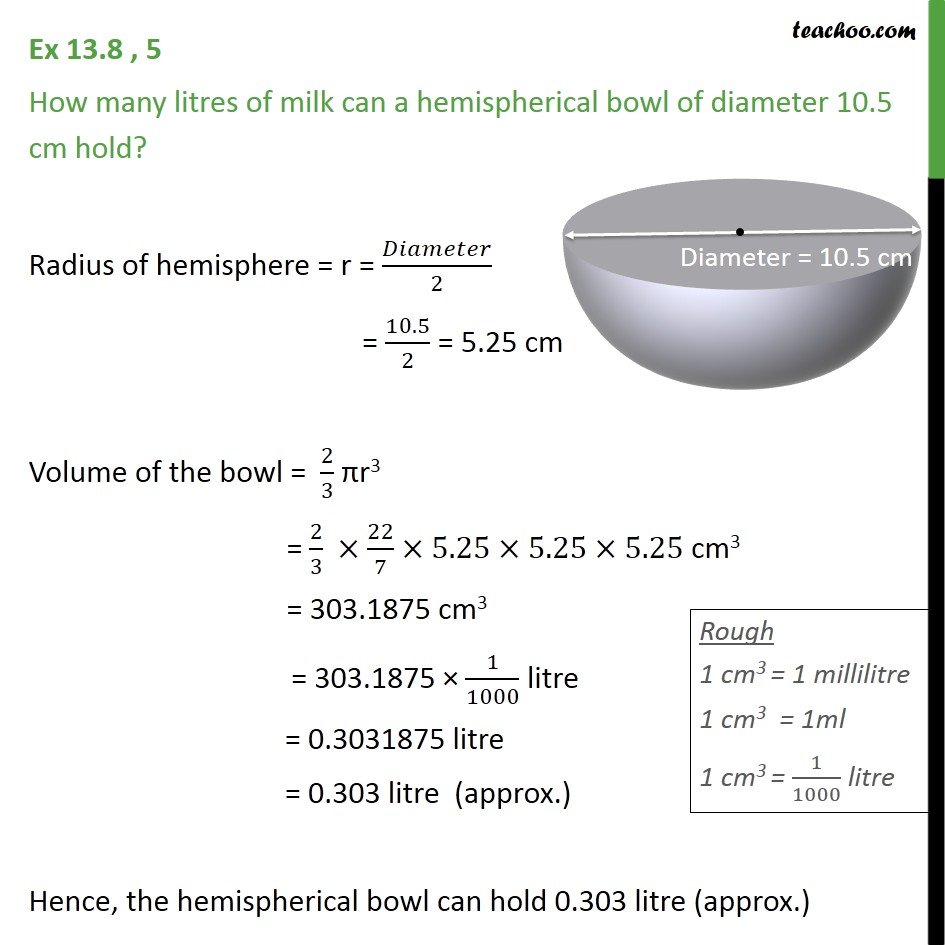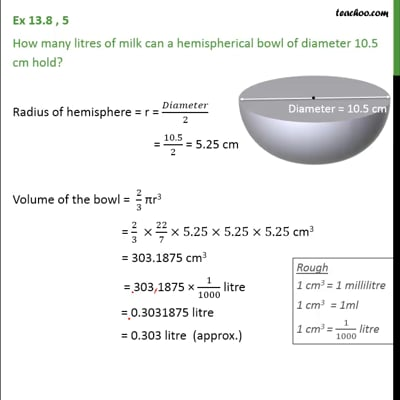Ex 13.8

Chapter 13 Class 9 Surface Areas and Volumes (Term 2)
Serial order wiseThis video is only available for Teachoo black users

### Transcript

Ex 13.8 , 5 How many litres of milk can a hemispherical bowl of diameter 10.5 cm hold? Radius of hemisphere = r = /2 = 10.5/2 = 5.25 cm Volume of the bowl = 2/3 r3 = 2/3 22/7 5.25 5.25 5.25 cm3 = 303.1875 cm3 = 303.1875 1/1000 litre = 0.3031875 litre = 0.303 litre (approx.) Hence, the hemispherical bowl can hold 0.303 litre (approx.)